# Comber Machine Production Calculation

Comber Machine Production Calculation
(With a practical experience)AGM,
M.Hossain Cotton Spinning Mills Ltd
Email: sebabd@ymail.com

### Comber machine and combing process:

Comber is a machine used in spinning mill by which fibre are combed and the action is called combing. Combing is a very important process that makes the difference between an ordinary yarn and a quality yarn. Combing operation carried out between carding and draw frame. In order to improve the quality of the sliver coming operation is carried out. By combing operation fiber become straight and parallel so that yarn become even and lustrous. Combed sliver has a better luster compared with carded sliver because of the improved fiber alignment. The materials extracted from the feed lap in the combing process are called noil. Noil contains short fibers, neps, and impurities. In this article I will give some calculations of comber machine which frequently do in my spinning mill.

Problem 1:

Find out the production/day a comber in tons from the following particulars:

Nips/min   : 350
Feed/Nips   : 7.5 mm
Noil extraction: 18%,
Lap weight : 1200 grains/yd and
Efficiency   : 85%.

Solution:

Given data,
Nips/min     : 350
Feed/Nips    : 7.5 mm
Noil extraction: 18%
: (100-18)/100
: 0. 82

Lap weight  : 1200 grains/yd
: 71.10 gm/m

Efficiency : 85% = 0.85

So, Production/day: ?

Comber production/shift: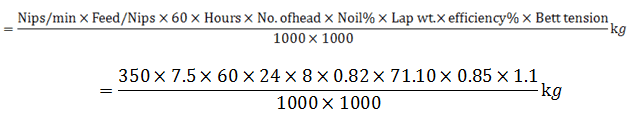= 1648.45 kg (Ans)

Problem 2:

Calculate the production in kg/shift of a comber machine having following particulars:

Lap count = 100 gram/meter
Feed/nip = 5mm
Nips/min = 350
Noil extraction =14% and
Efficiency = 90%.

Solution:

Given data,
Lap count = 100 gram/meter
Feed/nip = 5mm
Nips/min = 350
Noil extraction =14%
= (100-14)/100
= 0.86
Efficiency = 90%
= 0.90
Production/shift = ?

Comber production/shift: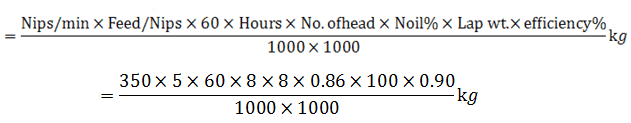= 520.13 kg (Ans)

Problem 3:

Calculate production of a comber machine in kg/shift, where -lap weight = 70ktex, feed/nip = 4.7 mm, nips/min = 380, number of head = 8, noil extraction =14% and efficiency = 90%.

Solution:

Given data,
Lap wt. = 70ktex = (70 x 1000) tex = 70 gm/m
Feed/nips    = 4.7 mm
Nips/min     = 380
Noil extraction= 14% = (100-14)/100 = 0.86
Efficiency         = 90% = 0.90

Production/Shift=?

Comber production/shift: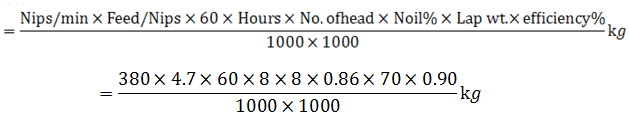= 371.58 kg (Ans)

Problem 4:

A spinning mill is run with 20 comber machine which process cotton fiber upto staple length 1.125 inches. Parameters of each machine are: nips/min =550, feed/nip = 5.8 mm, no. of head per machine = 8, lap weight = 800 gr/yd, waste extraction = 15%, efficiency = 80% . Find out the production in kg/shift of those combers?

Solution:

Given data,
Nips/min     = 550
Feed/nips    = 5.8 mm
Lap wt.      = 800 gr/yd = 731.52 gr/m = 47.402 gm/m
Noil extraction =15% = (100-15)/100 = 0.85
Efficiency    = 80% = 0.80

So, Production/Shift =?

Comber production/shift: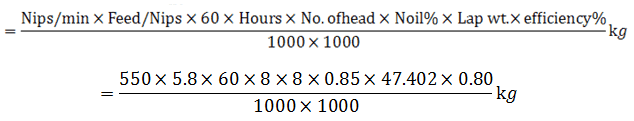= 394.845 kg

1 comber production/Shift = 394.845 kg

So, 20 comber production/shift = (394.845 x 20) kg = 7896.90 kg (Ans)

Problem 5:

The cylinder of a 6 head comber is running at a speed of 150 nips per minute and each nip feeds 0.185″ lap. The hank of lap is 0.0166. Calculate the production of comber in hours at 75% efficiency and 12% waste.

Solution:

Given data,
Nips/min     = 150
Feed/nips    = 0.185″
= 0.185 x 25.4) mm
= 4.7 mm

Lap hank     = 0.0166
= 32.53 gm/yd
= 29.745 gm/m

Noil = 12%
= (100-12)/100
= 0.88

Efficiency    = 75% = 0.75
So, production/hr =?

Comber production/hr: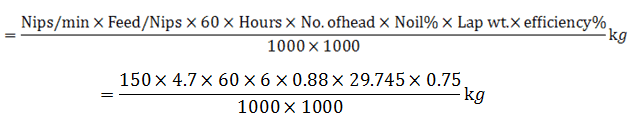= 4.982 kg/hr (Ans)

Problem 6:

Find out the production/shift/comber in kgs. (Assume necessary parameters)

Solution:

Let,
Nips/min               = up to 400 = 350
Feed/Nips             = 4.2 to 5.6 mm = 5.2 mm
Lap wt.                 = 45 – 80 gm/m = 80 gm/m
Noil                      = 10 – 30% = 15%
Efficiency             = up to 96% = 85% = 0.85
So, Production/Shift =?

Comber production/shift: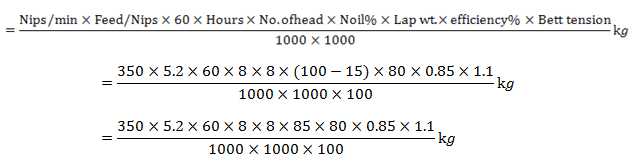= 444.35 kg.(Ans)

Problem 7:

Calculate the production per day of a combing machine when-

Nip/min      = 220
Feed/nip      = 7mm
Lap weight = 1000 grains/yd
Efficiency    = 90%
Noil            = 15%

Solution:

Given that,
Nip/min = 220
Feed/nip = 7mm
Feed lap weight = 1000 grains/yd
= 64.80 grains/yd
= 59.227 gm/m
Efficiency = 90% = 0.90
Noil % = 15% = (100-15)/100 = 0.85

Production/Day: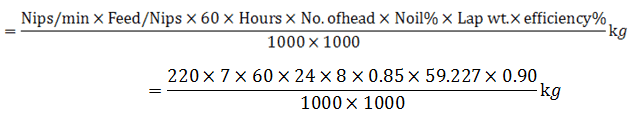= 803.81 kg (Ans)

Problem 8:

To calculate the production of comber machine of 8 hours shift. Using following particular?

Lap Hank              = 70 k Tex
Nips / Min.           =   400
Feed / Nip            =   5 mm
Bett Tension         =   10 %
Noil %                  =    16 %
Efficiency (in %)   =    85 %
Shift Time            =   8 Hours

Calculate:
i) Lap hank or lap wt.
ii) Bett Tension
iii) Feed/min
iv) Production/Shift

Solution:

i) Lap wt. = 70KTex

= 70 x 1000 Tex

…..70 x 1000
= ———————— gm/m
………1000

= 70 gm/m (Ans)

ii) Bett tension = 10%

…………………………………Bett Tension in %
Bett Tension = 1 + ———————–
…………………………………………100

…………..10
= 1 + ——-
………….100

= 1 + 0.10
= 1.1 (Ans)

iii) Feed/min

Given data,
Feed/nip = 5 mm
Nips/min = 400

So, Feed/min = Feed/nip x nips/min (mm)
= 5 x 400 (mm)
= 2000 mm
= 2 mtr (Ans)   [1000 mm = 1meter]

iv) Production calculation:

Given data,
Lap wt. = 70 gm/m
Feed/min  = 2 m
Bett Tension   = 1.1
Noil% = 16% = (100-16)/100 = 0.84
Efficiency (%) = 85% = 0.85
Time    = 8 hrs

So, Production/Shift=?

Production/Shift: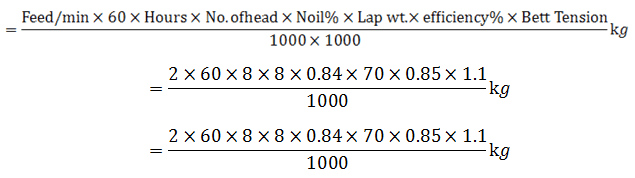= 422.23 kg (Ans)

Problem 9:

Calculate the finish time a lap if a combing machine is running with following datas:

Lap length = 130 yds
Lap roller speed = 2.25 rpm
Circumference = 9 inch
Efficiency (%) = 95%

Solution:

Given data,
Lap length             = 130 yds
Lap roller speed    = 2.25 rpm
Circumference       = 9 inch
Efficiency (%)         = 95% = 0.95

So, lap finish time =?

Feed lap length = Lap roller speed x Circumference x efficiency%
= 2.25 x 9 x 0.95 inch/min
= 19.237 x 0.0254 m/min
= 0.4886 x 60 m/hr
= 29.317 m/hr.

……………………………….Total lap length
Lap finish time = —————————–
……………………………….Feed lap length

….130 x 0.9144
= —————— hr
………..29.317

= 4.054 hr (Ans)

Or,

Feed lap length = Lap roller speed x Circumference x Efficiency%
= 2.25 x 9 x 0.95 inch/min
= 19.238 inch/min

…..19.238 x 60
= —————– yd/hr
………….36

= 32.062 yd/hr.

………………………………Total lap length
Lap finish time = —————————–
………………………………Feed lap length

………130
= —————– hr
……32.062

= 4.054 hr (Ans)

Problem 10:

Find out the production constant and production/shift in a comber machine as per following parameters:

Coiler calendar roller speed    = 125 rpm
Delivery sliver wt.                    = 70 gr/yd
Efficiency (%)                          = 95%.

Solution:

Given data,
Coiler calendar roller speed (N) = 125 rpm
Roller radius (r)                        = 1.25 ″
Delivery sliver wt.                    = 70 gr/yd
Efficiency (%)                          = 95% = 0.95

So, m/c constant                     =?
Production/shift                      = ?

We know that,

Production constant = Circumference of coiler calendar roll

……Π x 2r x N
= ———————— yd/min
………..36

……3.14 x 2 x 1.25 x 125
= ——————————–yd/min
………………….36

= 27.256 yd/min (Ans)

Production/shift: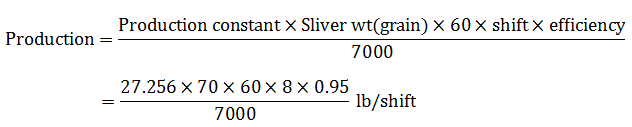= 124.29 lb/shift (Ans)

= 56.38 kg/shift (Ans)

Or,

Given data,

Coiler calendar roller speed (N)  = 125 rpm
Roller radius (r) = 1.25 rpm= (1.25 x 0.0254) m = 0.03175 m
Delivery sliver wt. = 70 gr/yd = 4.147 gm/m
Efficiency (%) = 95% = 0.95

So, m/c constant                       =?

Production/shift                       = ?

Production constant = π x 2r x N
= 3.14 x 2 x 0.03175 x 125
= 24.923 (Ans)

Production/shift: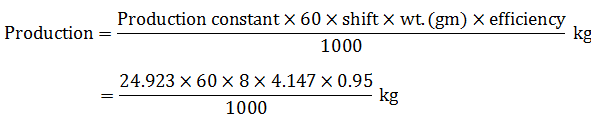= 47.13 kg/shift (Ans)

Problem 11:

Find the production/hr a comber machine from the following parameters:

1. Feed/Nip = 0.55″
2. Nip/Min = 140
3. Feed lap wt. = 1.6 ozs
4. No of head = 6
5. Wastage/Noil= 18%
6. Efficiency = 95%.

Solution:

Given data,

i) Feed/Nip = 0.55″

= (0.55 x 0.0254) m

= 0.01397 m

ii) Nip/Min = 140

iii) Feed lap wt.= 1.6 ozs

……1.6
= —— lbs [16 ozs = 1 lbs]
……16

= 0.1 lbs

= (0.1 x 453.6) gm [453.6 gm = 1 lbs]

= 45.36 gm

iv) No. of head     = 6

v) Wastage/Noil= 18%
= (100-18)/100
= 0.82

vi) Efficiency = 95% = 0.95

Production/hr =?

Production/hr: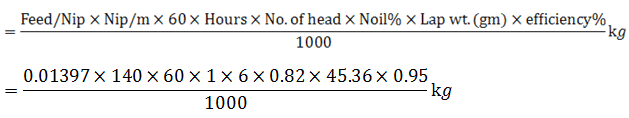= 24.879 kg (Ans)